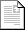ASEE NCS Conference 2019

Full Program »
 PDF Filepdf704KB

The value of lumped parameter analysis in introductory fluid mechanics courses

In virtually every introductory fluid mechanics book, the momentum principle (the combination of Newton's 2nd Law of Motion and Reynold's Transport Theorem) is introduced in distributed parameter integral form. This is not how the coservation of energy, conservation of mass nor the 2nd Law of Thermodynamics is introduced in the prior course. The goal of this paper is to show the utility of such a formulation that would serve as a better bridge between thermodynamics and introductory fluid mechanics courses.

Chris Kobus
Oakland University
United States